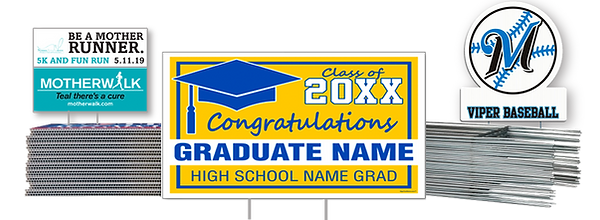top of page1/4 COROPLAST, OUTDOOR, INDOOR YARD SIGNS WITH STEP STAKE.  18 X 24"

PRINTED 1 SIDE

5 = \$20.00 EA

10 = \$16.00

30 = \$14.50

50 = \$12.75

100 = \$10.75

150 = \$9.75

200 = \$8.75

PRINTED 2 SIDES

5 = \$30.00 EA

10 = \$25.00

30 = \$19.50

50 = \$16.75

100 = \$13.75

150 = \$11.75

200 = \$  9.75

bottom of page